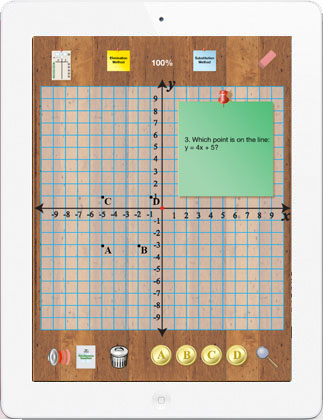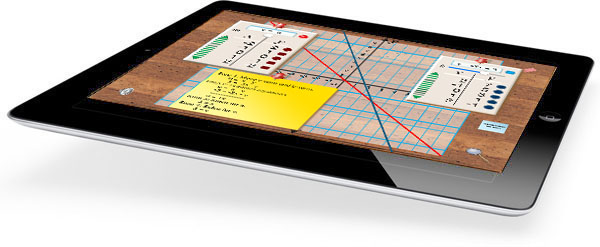Discover the Substitution and Elimination Methods for Solving Simultaneous Equations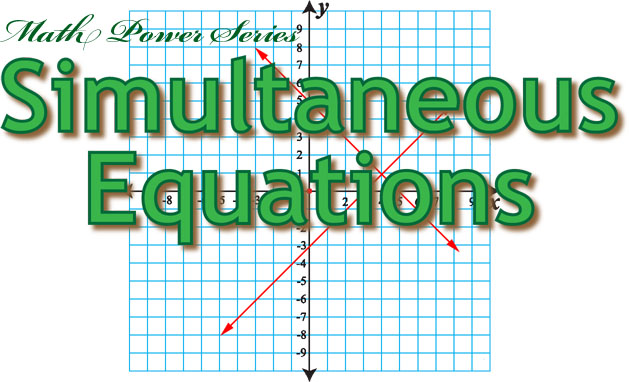Simultaneous Equations are a set of equations with two or more variables for which there are values that can satisfy all the equations simultaneously. The Simultaneous Equations app is an interactive tool designed to help teachers introduce the concepts related to simultaneous equations and demonstrates two methods for solving them. It provides students with an interactive learning device to help them better understand these important concepts. While using the app students set up the definitions of two lines. The lines are plotted on a coordinate grid. The coordinate pair for the intersection of the two lines represents the solution for the simultaneous equations. The Elimination and Substitution Methods are demonstrated. Designed in California by Dr. Fred Ventura, Simultaneous Equations is one of the apps in the Math Power Series. Simultaneous Equations turns your iPad into a mathematical playground for introducing the coordinate plane, ordered pairs, slope, y-intercept, and the graphical, substitution and elimination methods for solving simultaneous equations.

 Topics Activities Support Materials Equations in Slope Intercept Form Parallel Lines Slope and y-intercept Scale Graphing Simultaneous Equations Substitution Method Elimination Method Dynamically Illustrated Equations Exploratory Activities Student-Centered Learning iPad App Website

The primary objective for this app is to provide a method for students to visualize simultaneous equations graphed on a coordinate grid and to learn the substitution and elimination methods for finding a solution algebraically. Tap the table icon and begin defining an equation by using a slider to specify the slope and y-intercept of a line. Tap the plot button to graph the equation. Use the same procedure to define a second equation. Tap either the substitution or elimination icon to open a note and see the algebraic steps involved in finding the solution. Tap the question mark icon to start the quiz and measure a students understanding using randomly generated problems. Tap the notebook icon to begin reading an illustrated lesson that includes information about real-world applications for simultaneuos equations.

 Simultaneous Equations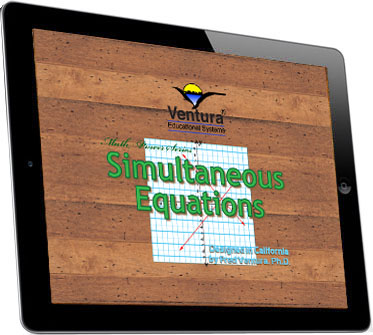The Importance Learning to Solve Simultaneous Equations The mathematics curriculum traditionally has been designed to provide students with the opportunity to develop skills and acquire knowledge that would be important in adulthood and to prepare sutdents to enter careers that would not require a high level of mathematical ability. Today the context of mathematics instruction needs to be much broader. The accelerated pace at which modern society produces technological change requires that high school graduates be prepared for careers in qhich mathematics will be very important. In order to be successful in many careers students will need to be confident in their mathematical abilities. They will need to be able to solve problems, to communicate mathematical ideas and to think logically. Simultaneous Equations turns your iPad into a power tool for teaching the concepts related to solving simultaneous equations using the graphical, substitution and elimination methods. Use Simultaneous Equations to create a presentation while illustrating and discussing the key principles of analytic geometry. Simultaneous Equation is perfect for algebra students in middle school and high school classrooms. Enrich your mathematics curriculum with the power of Simultaneous Equations! Simultaneous equations are a system of equations where a solution can be found for two variables so that when the values are substituting into the equations, both equations are true. Finding the solution involves working with two equations simultaneously. The graph of any equations shows all the values for which the equation is true. In solving simultaneous equations, the goal is the find the values for the variables where both equations are true. Simultaneous equations are used to solve many everyday problems. Here is a list of some applications where being able to solve simultaneous equations is helpful: Rate, Distance and Time problems Finding the Best Rate Plan Choosing the Best Loan Investing Mixing Chemical Compounds and many other problems... The Simultaneous Equations app creates a basic set of learning experiences to help students better understand of methods for solving simultaneous equations. Coordinate geometry bridges algebra and geometry. Learning the Substitution and Elimination Methods for Solving Simultaneous Equations is fundamental to success in higher mathematics. Main topics: Equations in Slope Intercept Form Parallel Lines Slope and y-intercept Scale Substitution Method Elimination Method

Simultaneous Equations - A Powerful Teaching Tool

 \$1.99
 Simultaneous Equations Simultaneous Equations is an effective way to introduce methods for finding solutions to simultaneous equations. Tap icons and use simple gestures to define lines using the slope/intercept format. Student are able to manipulate the lines and instantly see the effect graphically. Tap a note icon to instantly see the algebraic solutions using either the substitution or elimnation methods. Interactions are described mathematically, for example, use a slider to change the slope of a line and instantly see the result graphically and algebraically.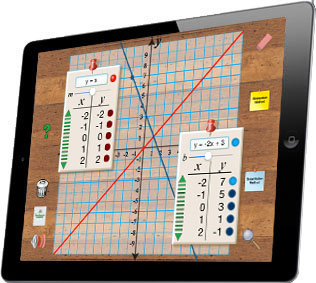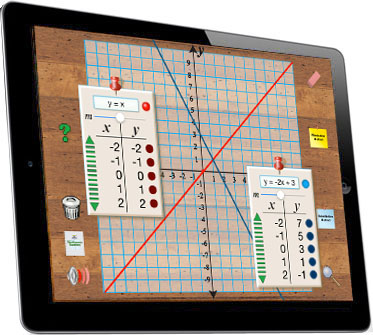Interactive Inquiry Tap areas of the screen to access the special tools for exploring simultaneous equations. Manipulate the slope and y-intercept of two lines and instantly see the effects graphically. Tap the yellow or blue post-it icon to see a step-by-step explanation of the Elimination or Substitution Method for finding the solution.Step-by-Step Solutions Tap a note icon to see step-by-step solutions for the equations defined by the student.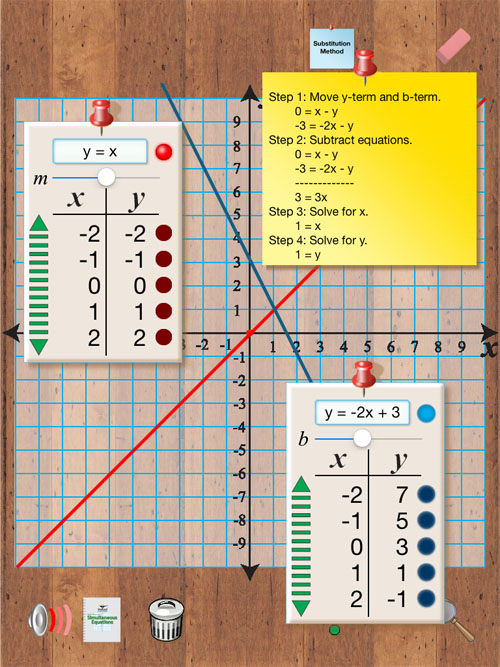Real Learning That Is Fun and Creative In addition to all the math that students can learn using Simultaneous Equation, it is also a tool for just having fun and being creative using mathematics. Let your students develop an intuitive understanding of how to find a solution to a set of equations by letting them play with the app in a creative way. Here’s an idea for a cooperative learning game that you can use if you have two students and two iPads.   Procedure: Two players sit facing each other but can see the other players iPad.   Player 1 picks a point on the graph, for example, (3,-3). The player writes the coordinate pair for the point on a index card and gives it face down to player 2. Player 2 also picks a point on the graph, for example, (-3,2). The player writes the coordinate pair for the point on an index card and gives it face down to player 1. The first player to define a set of two lines that intersect at the point written on his or her card wins the round.